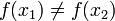# Injective function

In mathematics, an injective function or one-to-one function or injection is a function which has different output values on different input values: f is injective if$x_1 \neq x_2$ implies that$f(x_1) \neq f(x_2)$.

An injective function f has a well-defined partial inverse f − 1. If y is an element of the image set of f, then there is at least one input x such that f(x) = y. If f is injective then this x is unique and we can define f − 1(y) to be this unique value. We have f − 1(f(x)) = x for all x in the domain.

A strictly monotonic function is injective, since in this case x1 < x2 implies that f(x1) < f(x2) (if f is increasing) or f(x1) > f(x2) (if f is decreasing).Some content on this page may previously have appeared on Citizendium.